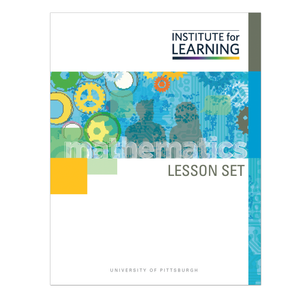# Investigating Congruence in Terms of Rigid Motion (Geometry)

Regular price \$6.25 \$0.00 Unit price per

In this lesson set, students will formalize their understanding of the rigid motions translation, rotation, and reflection. Congruence will be defined through rigid motions, and criteria for triangle congruence will be proved and applied.

Through engaging in the lessons in this lesson set, students will:

• formalize their definition of rigid motions in terms of the movement of figures in the coordinate plane;
• describe and predict the transformation of shapes undergoing a sequence of rigid motions;
• use the definition of congruence in terms of rigid motion to prove the criteria for triangle congruence; and
• apply the criteria for triangle congruence in solving problems and proving theorems.

Standards: G-CO.6, G-CO.7, G-CO.8